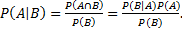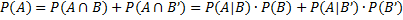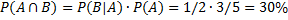# Basic Probability Concepts

Definition 1: Typically in the field of statistics we study data that results from experiments. An experiment can be considered to be a series of trials, each with a particular outcome. An event is a collection of outcomes corresponding to some result in the experiment. The number of outcomes in event E (i.e. the number of elements in set E) is written as |E|. The set of all possible outcomes is called the sample space, often designed  S. An event is then simply a subset of the sample space. The probability P(E) of the event E is |E| / |S|, assuming S is not empty.

Example 1: Consider the simple experiment of tossing a coin twice. What is the probability that the coin comes up heads both time?

The sample space S = {HH, HT, TH, TT} and the required event E = {HH}. Thus the probability that the coin is heads both times is P(E) = |E| / |S| = ¼, or 25%.

Observation: We now state the fundamental properties of probability, using the usual set notation.

Property 1:

1. 0 ≤ P(A) ≤ 1
2. P(Ø) = 0
3. P(S) = 1
4. P(A′) = 1 – P(A), where A′ = S – A
5. P( B) = P(A) + P(B) – P(A ∩ B)

Proof: Simple consequences of Definition 1.

Example 2: Consider the experiment of drawing one card from a standard deck of 52 cards. What is the probability of drawing either a spade or face card?

There are 13 spades and 12 face cards, but 3 of these face cards are also spades, which we should not count twice. Thus, there are 13 spades and 9 non-spade face cards for a total of 22 cards out of 52. The probability is therefore 22/52. We now show how to calculate the result using Property 1e.

Let A = the event that a spade is drawn and B = the event that a face card (King, Queen or Jack) is drawn. P(A) = 13/52, P(B) = 12/52 and P(A ∩ B)= 3/52. Thus the probability of drawing either a spade or face card is P( B) = P(A) + P(B) – P(A ∩ B) = 13/52 + 12/52 – 3/52 = 22/52.

Definition 2: The probability that an event A occurs assuming that event B occurs is called the conditional probability of A given B and is denoted P(A|B).

Observation: By Definitions 1 and 2

Property 2:

1. P(A|B) ∙ P(B) = P(AB) = P(B|A) ∙ P(A)
2. P(A|B) = P(B|A) ∙ P(A) / P(B) called Bayes’ Theorem
3. P(A) = P(A|B) ∙ P(B) + P(A|B′) ∙ P(B′) called the Law of Total Probability

Proof: The first assertion is a restatement of the last observation. The second assertion is a consequence of two applications of the first sinceWe now prove the third assertion. Since A  = (AB) ∪ (AB′), by Properties 1b and 1e,

Now by Property 2a and 2b,which proves the third assertion.

Example 3: Consider the experiment of picking two balls at random without replacement from a bag which contains 3 reds and 2 blacks. What is the probability that both balls are red?

Let A = a red ball is taken on the first draw and B = a red ball is taken on the second draw. The probability that the first draw is red is P(A) = 3/5. The probability that the second draw is red given the first draw is red is P(B|A) = 2/4 = ½. From Property 2a, we see that the probability that both draws are red isDefinition 3: Two events A and B are independent if P(AB) = P(A) ∙ P(B)

Property 3: Two events A and B are independent if and only if P(A) = P(A|B)

Proof: A and B are independent if and only if P(A∩B) = P(A) ∙ P(B), which by Property 2a is true if and only if P(A|B) ∙ P(B) = P(A) ∙ P(B), which in turn is true if and only if P(A|B) = P(A).

ObservationA and B are independent if B’s occurring (or not occurring) has no influence on A’s occurring, i.e. it doesn’t increase or decrease the probability of A occurring. By Property 3, A and B are independent if any only if P(B|A) = P(B), and so it also follows that if A and B are independent then A’s occurring has no influence on B’s occurring either.

Example 4: Repeat the experiment from Example 3, but this time we put the ball picked on the first draw back in the bag before drawing a second ball (i.e. sampling with replacement).

Since P(B|A) = 3/5 = P(B), A and B are independent, it follows that

P(AB) = P(A) ∙ P(B) = 3/5 ∙ 3/5 = 36%.

Example 5: You have two bags, one containing 3 red and 2 black balls, the other containing 1 red, 1 blue and 2 black balls. You pick a bag at random and then pick a ball from that bag at random. What is the probability that the ball picked is red?

Let A = event that the first bag is picked and let B = event that a red ball is drawn. By Property 2c,

P(B) = P(B|A) ∙ P(A) + P(B|A′) ∙ P(A′) = .6(.5) + .25(.5) = 42.5%.

Example 6: Suppose you role a die 12 times. What is the probability that the number 1 will not appear on any of the throws? What is the probability that the number 1 will appear on at least one of the 12 throws?

The 12 throws represent 12 independent events. The probability of throwing a 1 on any single trial is 1/6 and so the probability of not throwing a 1 on any single trial is 1 – 1/6 = 5/6 (by Property 1d). Thus the probability of not throwing a 1 on any of the 12 throws is (5/6)12 = 11.2% (by Definition 3).

The probability that the number 1 will appear at least once is simply 1 – 11.2% = 88.8% (by Property 1d). This is equivalent to 1 – (1 – 1/6)12.

# Personal Career & Learning Guide for Data Analyst, Data Engineer and Data Scientist

## Applied Machine Learning & Data Science Projects and Coding Recipes for Beginners

A list of FREE programming examples together with eTutorials & eBooks @ SETScholars

# Projects and Coding Recipes, eTutorials and eBooks: The best All-in-One resources for Data Analyst, Data Scientist, Machine Learning Engineer and Software Developer

Topics included: Classification, Clustering, Regression, Forecasting, Algorithms, Data Structures, Data Analytics & Data Science, Deep Learning, Machine Learning, Programming Languages and Software Tools & Packages.
(Discount is valid for limited time only)`Disclaimer: The information and code presented within this recipe/tutorial is only for educational and coaching purposes for beginners and developers. Anyone can practice and apply the recipe/tutorial presented here, but the reader is taking full responsibility for his/her actions. The author (content curator) of this recipe (code / program) has made every effort to ensure the accuracy of the information was correct at time of publication. The author (content curator) does not assume and hereby disclaims any liability to any party for any loss, damage, or disruption caused by errors or omissions, whether such errors or omissions result from accident, negligence, or any other cause. The information presented here could also be found in public knowledge domains.`

# Learn by Coding: v-Tutorials on Applied Machine Learning and Data Science for Beginners

Please do not waste your valuable time by watching videos, rather use end-to-end (Python and R) recipes from Professional Data Scientists to practice coding, and land the most demandable jobs in the fields of Predictive analytics & AI (Machine Learning and Data Science).

The objective is to guide the developers & analysts to “Learn how to Code” for Applied AI using end-to-end coding solutions, and unlock the world of opportunities!